# 平面图形的面积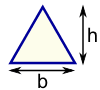三角形 面积 = ½ × 底 × 高 b = 底 h = 垂直高度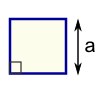正方形 面积 = a2 a = 边长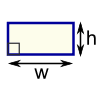矩(长方)形 面积 = 宽 × 高 w = 宽 h = 高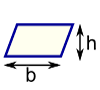平行四边形 面积 = b × h b = 底 h = 垂直高度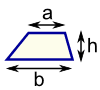梯形 面积 = ½（a + b） × h h =垂直高度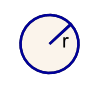圆形 面积 = π × r2 周长 = 2 × π × r r = 半径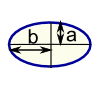椭圆形 面积 = πab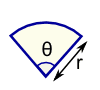扇形 面积 = ½ × r2 × θ r =半径 θ=角度（以弧度计算）
 注意：h 与 b 成直角：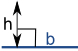### 例子：这个矩形的面积是什么？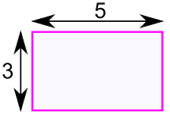w = 宽
h = 高

### 例子：这个圆形的面积是什么？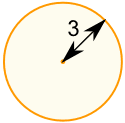面积 = π × r2 = π × 32 = π × （3 × 3） = 3.14159 ... × 9 = 28.27 （算到小数点后第 2 位）

### 例子：这个三角形的面积是什么？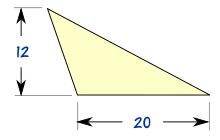### 山姆为这草地剪草的收入是多少钱：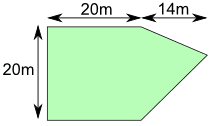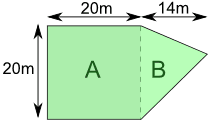A 部份是个正方形：

A 的面积 = a2 = 20米 × 20米 = 400平方米

B 部份是三角形。横看它的底是 20米而高是 14米。

B 的面积 = ½ b × h =½ × 20米 × 14米 = 140平方米